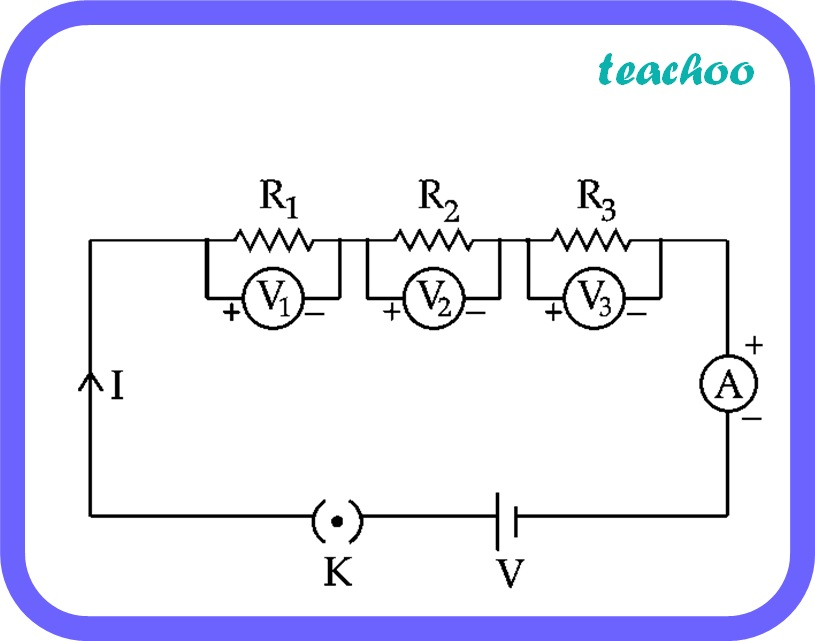Past Year - 5 Mark Questions

Class 10
Chapter 12 Class 10 - Electricity

## Establish a relationship to determine the equivalent resistance R of a combination of three resistors having resistances R 1 , R 2 and R 3 connected in series. Calculate the equivalent resistance of the combination of three resistors of 2 Ω, 3 Ω and 6 Ω joined in parallel.

(i)

An applied potential V produces current I in the resistors R1, R2 and R3, causing a potential drop V1, V2 and V3 respectively through each resistor.Total Potential, V = V 1 + V 2 + V 3

Let R be the equivalent resistance.

According to ohm’s law,

V = IR

Therefore,

• V 1 = IR 1
• V 2 = IR 2
• V 3 = IR 3

Thus, V = IR 1 + IR 2 + IR 3

IR = I(R 1 + R 2 + R 3

R =  R 1 + R 2 + R 3

This proves that equivalent Resistance R is the sum of resistances R 1 , R 2 , R 3

(ii)

Three resistors 2 Ω, 3 Ω and 6 Ω, are connected in parallel combination.

Let R be the equivalent resistance.

1 / R = 1 / 2 + 1 / 3 + 1 / 6

= ( 3 + 2 + 1 ) / 6

= 6 / 6

Therefore, R = 1 ohm

Learn in your speed, with individual attention - Teachoo Maths 1-on-1 Class